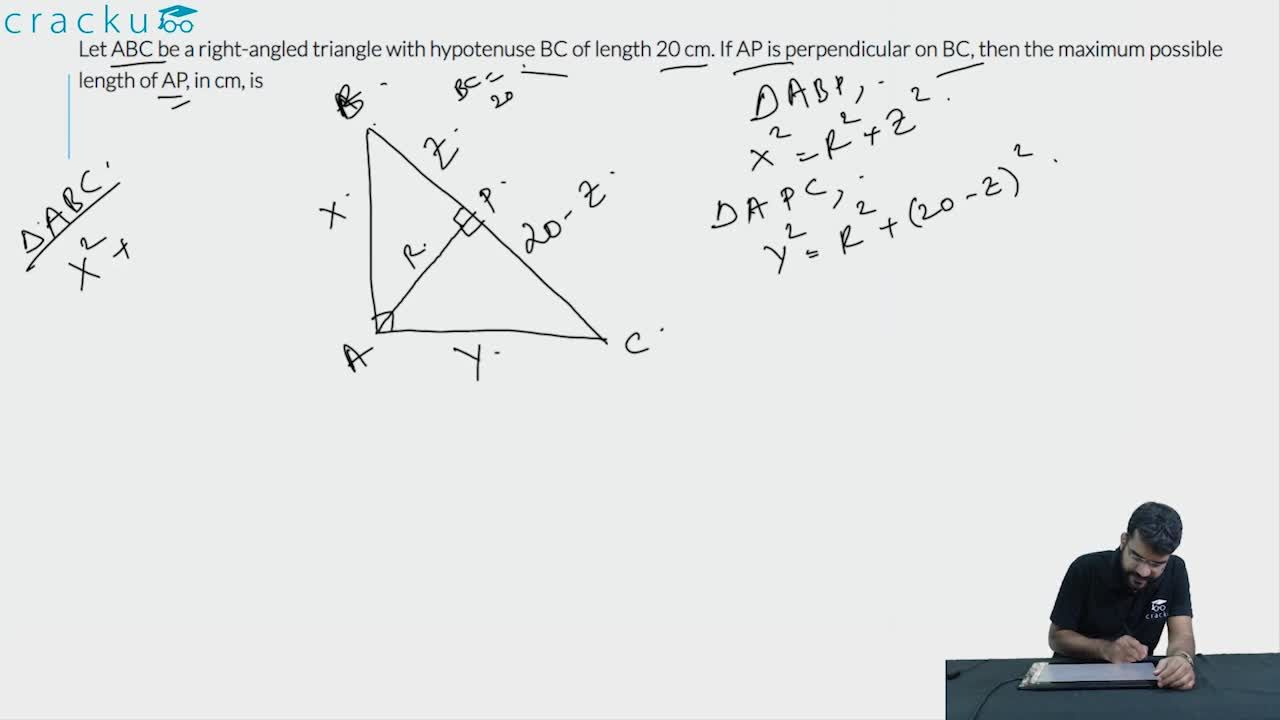Question 11

# Let ABC be a right-angled triangle with hypotenuse BC of length 20 cm. If AP is perpendicular on BC, then the maximum possible length of AP, in cm, is

Solution

Let p be the length of AP.

It is given that $$\angle\ BAC\ =90\$$ and $$\angle\ APC\ =90\$$

Let $$\angle\ ABC\ =\theta\$$, then $$\angle\ BAP\ =90-\theta\$$ and $$\angle\ BCA\ =90-\theta\$$

So $$\angle\ PAC\ =\theta\$$

Triangles BPA and APC are similar

$$p^2=x\left(20-x\right)$$

We have to maximize the value of p, which will be maximum when x=20-x

x=10

### View Video Solution